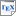[CDKM11] T. Chen, M. Diciolla, M. Kwiatkowska and A. Mereacre. Time-Bounded Verification of CTMCs against Real-Time Specifications. In Proc. 8th International Conference on Formal Modeling and Analysis of Timed Systems (FORMATS'11), volume 6919 of LNCS, pages 26-42, Springer. September 2011. [pdf] [bib] Downloads:pdf (295 KB)bib Notes: The original publication is available at link.springer.com. Links: [Google] [Google Scholar] [CiteSeer] Abstract. In this paper we study time-bounded verification of a finite continuous-time Markov chain (CTMC) C against a real-time specification, provided either as a metric temporal logic (MTL) property φ, or as a timed automaton (TA) A. The key question is: what is the probability of the set of timed paths of C that satisfy φ (or are accepted by A) over a time interval of fixed, bounded length? We provide approximation algorithms to solve these problems. We first derive a bound N such that timed paths of C with at most N discrete jumps are sufficient to approximate the desired probability up to ε. Then, for each discrete (untimed) path σ of length at most N, we generate timed constraints over variables determining the residence time of each state along σ, depending on the real-time specification under consideration. The probability of the set of timed paths, determined by the discrete path and the associated timed constraints, can thus be formulated as a multidimensional integral. Summing up all such probabilities yields the result. For MTL, we consider both the continuous and the pointwise semantics. The approximation algorithms differ mainly in constraints generation for the two types of specifications.